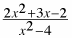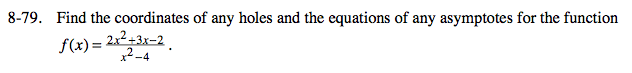### Home > PC > Chapter 8 > Lesson 8.2.1 > Problem8-79

8-79.

Find the coordinates of any holes and the equations of any asymptotes for the function f(x) =. Homework Help ✎Factor completely:

$f(x)=\frac{(2x-1)(x+2)}{(x-2)(x+2)}$

HOLES or VERTICAL ASYMPTOTES occur when the denominator = 0. In this case x = −2 is where a hole occurs because the factor divides evenly into the numerator. And x = +2 is a vertical asymptote because the factor does not divide evenly into the numerator.

Since the dominant term is 2x2 in the numerator and x2 in the denominator, y = 2 is the horizontal asymptote.